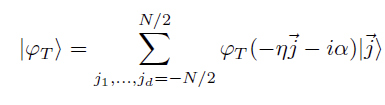# How to define a TT-cross implementation using ITensors without usage of for loops

Hello everyone I was trying to define the following ket state from a paper which has the following equation:where \varphi is a complex valued function, the only way I was able to produce this is by the usage of the following code for a 3d case:

i = Index(N,"i")
j = Index(N,"j")
k = Index(N,"k")
p = ITensor(i,j,k)
for iv in eachindex(p)
j = collect(Tuple(iv) .- N/2)
p[iv] = phi(-eta .*j .- im *alpha)
end


Is it possible to avoid the for loop and use the formalism of the ITensor to provide a TT-train approximation of such quantity?
For example if I use the tntorch python library I can use a lambda function to define it:

tn.cross(function=lambda x: x**2, tensors=[t])  # Compute the element-wise square of t using 5 TT-ranks

1 Like